# Formulas

A formula is a mathematical equation used to calculate a value. It can be entered directly in a cell or through the Formula Bar.

A formula can contain both mathematical expressions created by yourself and the ones already available in Spreadsheet (hereafter referred to as functions).

## Constructing a formula

• All formulas begin with the equal sign ( = ). The symbol tells Spreadsheet that the following numbers, operators, cells' references make up a formula;
• A formula that contains just numbers won't ever change the result value (e.g. formula =100/5+2 produces the result 22);
• A formula that contains cell or range references will change the produced result each time cells' values are changed (e.g. formula =A1+B1 produces the result that depends on the values of the cells A1 and B1);
• A formula can contain the following mathematical operators:
• "∗" (multiplication);
• "/" (division);
• "-" (subtraction).

### Order of operations

In case a formula uses more than one operator, there is a specific order that Spreadsheet will follow to perform the mathematical operations.

The order of operations is:

1. Calculations inside of parentheses;
2. Multiplications;
3. Divisions;
5. Subtractions.
• Any operation(s) contained in the parentheses will be carried out before the rest of the formula operations;
• Division and multiplication operations have equal importance, and after calculations in the parentheses are finished Spreadsheet performs these operations working from left to right in the equation;
• Addition and subtraction are also equal in the order of operations. The operation that goes first in the equation (left to right) will be carried out first.

## Entering formula

### Entering a formula in a cell

• Select the cell in which you want to enter a formula.
• Type the equal sign '=' to activate the Formula Window and type a formula.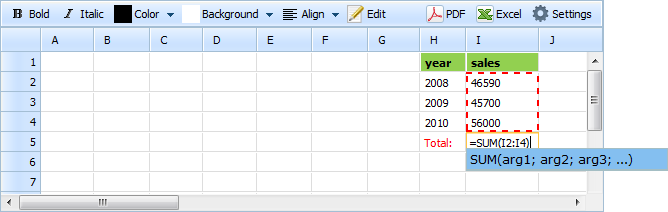When the formula is typed in and the ENTER button is pressed the result is immediately shown in the cell.

### Entering a formula through the Formula Bar

• Select the cell in which you want to enter a formula.
• Click on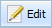to activate the Formula Bar and type a formula.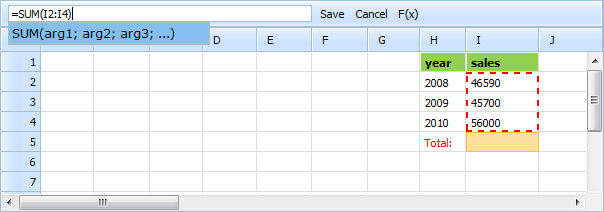• After you have completed the formula, press the ENTER button or click the Save button.

• To close the Formula Bar and cancel the currently typed formula click the Cancel button.

## Using functions in a formula

Generally, to add a function to a formula you need just type it according to the syntax shown in the table below (it concerns typing both in a cell and in the Formula Bar).

You can simply enter the first letter(s) of the desired function and the drop-down list, populated with the items that start from the specified letter(s), will appear.

### Formula bar

From the Formula Bar you also have the possibility to select a function from the Formula Palette without typing it out manually.

To use the Formula Palette act as it's stated below:

• click the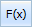button in the Formula Bar: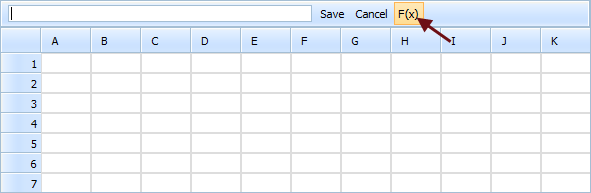• in the opened window select the desired function and click:

• the button Insert to enter a function and close the window;
• the button Cancel to cancel inserting and close the window.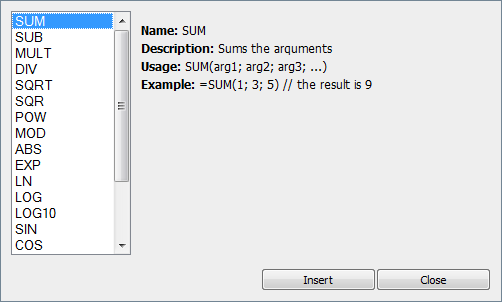## Cell references

You can use three types of cell references in Spreadsheet:

1. Relative
2. Absolute
3. Mixed

Let's suppose that you have a sheet similar to the one given below and need to copy the formula from the cell B1 (formula is '=A1*100') to the cell C3. The formula you will finally have in the cell C3 depends on the reference type you use in the cell B1.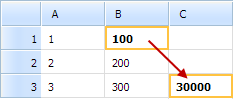Reference Type Formula in cell B1 Formula in cell C3 Calculated value in cell C3 Relative (default) =A1∗100 =B3∗100 30000 Absolute =\$A\$1∗100 =\$A\$1∗100 100 Mixed =\$A1∗100 =\$A3∗100 300 Mixed =A\$1∗100 =B\$1∗100 10000

## Function Cheat Sheet

 Function Details Syntax Example ABS Returns the absolute value of the argument ABS(arg1) ABS(-5) (returns 5) ACOS Returns the arc cosine (in radians) of the arqument ACOS(arg1) ACOS(1) (returns 0) ASIN Returns the arc sine (in radians) of the argument ASIN(arg1) ASIN(0) (returns 0) ATAN Returns the arctangent (in radians) of the argument ATAN(arg1) ATAN(1) (returns 0.78539816) COS Returns the cosine of the arqument COS(arg1) COS(0) (returns 1) DIV Divides the first argument by the second and subsequent arguments DIV(arg1; arg2; arg3; ...) DIV(I11;I9;I5) (equal to I11/(I9∗I5)) EXP Returns e raised to power of the argument EXP(arg1) EXP(2) (returns 7.3890561) LN Returns the natural logarithm of the argument LN(arg1) LN(7.3890561) (returns 2) LOG Returns the logarithm of the first argument to a base of the second argument LOG(arg1;arg2) LOG(32;2) (returns 5) LOG10 Returns the base-10 logarithm of the argument LOG10(arg1) LOG10(100) (returns 2) MOD Returns the remainder after the first argument is divided by the second argument MOD(arg1;arg2) MOD(10;4) (returns 2) MULT Multiplies the arquments MULT(arg1; arg2; arg3; ...) MULT(A2:D2;A6) PI Returns the mathematical constant called pi PI() PI() (returns 3.14159265) POW Returns the first argument raised to power of the second argument POW(arg1; arg2) POW(2;5) (returns 32) SIN Returns the sine of the argument SIN(arg1) SIN(0) (returns 0) SQR Returns the square of the arqument SQR(arg1) SQR(3) (returns 9) SQRT Returns the square root of the argument SQRT(arg1) SQRT(16) (returns 4) SUB Subtracts the second and subsequent arguments from the first argument SUB(arg1; arg2; arg3; ...) SUB(A10;B3;C3;D3) (equal to A10-B3-C3-D3) SUM Sums the arquments SUM(arg1; arg2; arg3; ...) SUM(A5;B6;C8) TAN Returns the tangent of the argument TAN(arg1) TAN(0) (returns 0)

Note, instead of digits used in the examples in the table above you can use cell or range references and vice versa.